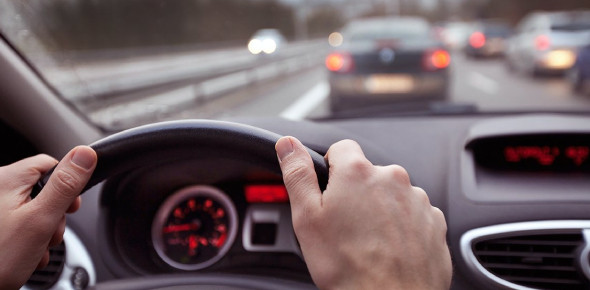# Speed Limit Test: Trivia Quiz!

10 Questions | Total Attempts: 136Settings.

• 1.
What is the speed limit for cars on a single carriageway?
• A.

40mph

• B.

60mph

• C.

70mph

• D.

50mph

• 2.
What is the speed limit for cars on a dual carriageway?
• A.

70mph

• B.

60mph

• C.

40mph

• D.

50mph

• 3.
What is the speed limit for cars towing caravans or trailers on a dual carriageway?
• A.

40mph

• B.

70mph

• C.

50mph

• D.

60mph

• 4.
What is the speed limit for cars in a built-up area?
• A.

15mph

• B.

25mph

• C.

30mph

• D.

20mph

• 5.
What is the speed limit for cars on the motorway?
• A.

60mph

• B.

65mph

• C.

70mph

• D.

75mph

• 6.
What is the speed limit for buses on a dual carriageway?
• A.

50mph

• B.

55mph

• C.

65mph

• D.

60mph

• 7.
What is the speed limit for buses in a built-up area?
• A.

30mph

• B.

25mph

• C.

35mph

• D.

40mph

• 8.
What is the speed limit for Motorhomes on a dual carriageway?
• A.

65mph

• B.

70mph

• C.

80mph

• D.

60mph

• 9.
What is the speed limit in a built-up area near a school?
• A.

30mph

• B.

25mph

• C.

20mph

• D.

15mph

• 10.
What is the speed limit for motorcycles on a dual carriageway?
• A.

65mph

• B.

80mph

• C.

60mph

• D.

70mph

Related TopicsBack to top